## Chebyshev Polynomial of the First Kind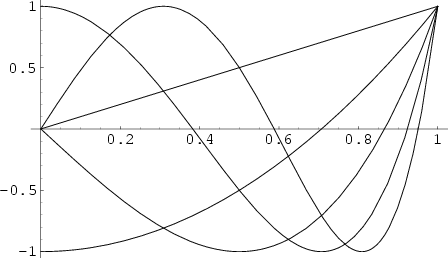A set of Orthogonal Polynomials defined as the solutions to the Chebyshev Differential Equation and denoted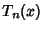. They are used as an approximation to a Least Squares Fit, and are a special case of the Ultraspherical Polynomial with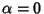. The Chebyshev polynomials of the first kindare illustrated above for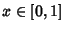and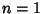, 2, ..., 5.

The Chebyshev polynomials of the first kind can be obtained from the generating functions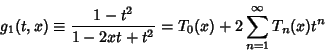(1)

and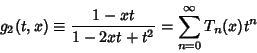(2)

for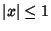and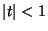(Beeler et al. 1972, Item 15). (A closely related Generating Function is the basis for the definition of Chebyshev Polynomial of the Second Kind.) They are normalized such that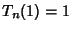. They can also be written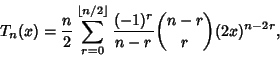(3)

or in terms of a Determinant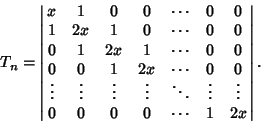(4)

In closed form,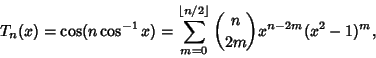(5)

where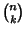is a Binomial Coefficient and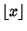is the Floor Function. Therefore, zeros occur when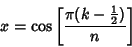(6)

for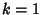, 2, ...,. Extrema occur for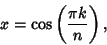(7)

where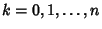. At maximum,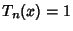, and at minimum,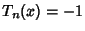. The Chebyshev Polynomials are Orthonormal with respect to the Weighting Function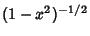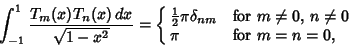(8)

where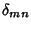is the Kronecker Delta. Chebyshev polynomials of the first kind satisfy the additional discrete identity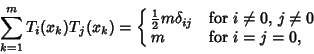(9)

where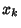for, ...,are thezeros of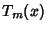. They also satisfy the Recurrence Relations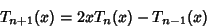(10)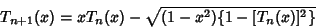(11)

for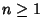. They have a Complex integral representation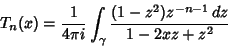(12)

and a Rodrigues representation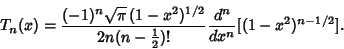(13)

Using a Fast Fibonacci Transform with multiplication law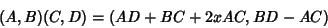(14)

gives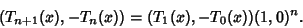(15)

Using Gram-Schmidt Orthonormalization in the range (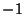,1) with Weighting Function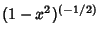gives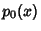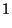(16)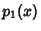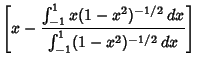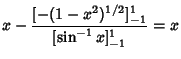(17)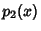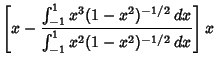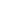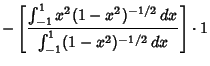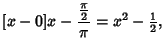(18)

etc. Normalizing such thatgives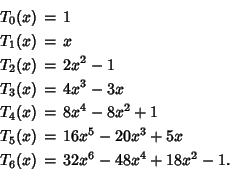The Chebyshev polynomial of the first kind is related to the Bessel Function of the First Kind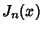and Modified Bessel Function of the First Kind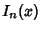by the relations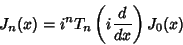(19)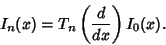(20)

Letting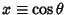allows the Chebyshev polynomials of the first kind to be written as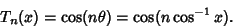(21)

The second linearly dependent solution to the transformed differential equation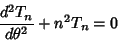(22)

is then given by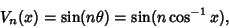(23)

which can also be written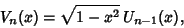(24)

where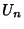is a Chebyshev Polynomial of the Second Kind. Note that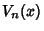is therefore not a Polynomial.

The Polynomial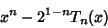(25)

(of degree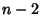) is the Polynomial of degree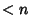which stays closest to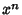in the interval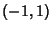. The maximum deviation is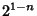at the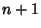points where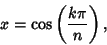(26)

for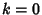, 1, ...,(Beeler et al. 1972, Item 15).

References

Abramowitz, M. and Stegun, C. A. (Eds.). Orthogonal Polynomials.'' Ch. 22 in Handbook of Mathematical Functions with Formulas, Graphs, and Mathematical Tables, 9th printing. New York: Dover, pp. 771-802, 1972.

Arfken, G. Chebyshev (Tschebyscheff) Polynomials'' and Chebyshev Polynomials--Numerical Applications.'' §13.3 and 13.4 in Mathematical Methods for Physicists, 3rd ed. Orlando, FL: Academic Press, pp. 731-748, 1985.

Beeler, M.; Gosper, R. W.; and Schroeppel, R. HAKMEM. Cambridge, MA: MIT Artificial Intelligence Laboratory, Memo AIM-239, Feb. 1972.

Iyanaga, S. and Kawada, Y. (Eds.). Cebysev (Tschebyscheff) Polynomials.'' Appendix A, Table 20.II in Encyclopedic Dictionary of Mathematics. Cambridge, MA: MIT Press, pp. 1478-1479, 1980.

Rivlin, T. J. Chebyshev Polynomials. New York: Wiley, 1990.

Spanier, J. and Oldham, K. B. The Chebyshev Polynomialsand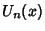.'' Ch. 22 in An Atlas of Functions. Washington, DC: Hemisphere, pp. 193-207, 1987.